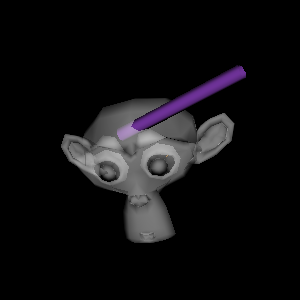# Getting Started#

Start by importing FURY.

```import numpy as np
from fury import window, actor, ui, io, utils
```

To import a model, use `io.load_polydata()`. Currently supported formats include OBJ, VTK, FIB, PLY, STL and XML.

Here we are going to use `suzanne` model used by Blender. You can find more detail about what is suzanne model here.

```from fury.data import fetch_viz_models
fetch_viz_models()
```

The models will be downloaded in `~/.fury/models` directory.

If you store the return value to `models`, you can find the detailed information about models in the dictionary. The directory of the `suzanne` model will be in `models`.

```models = fetch_viz_models()
print(models)
```

To get the path of suzanne

```import os
path_suzanne = os.path.join(models, 'suzanne.obj')
print(path_suzanne)
```

Now we can include the `suzanne` model used by Blender:

```suzanne = io.load_polydata(path_suzanne)
suzanne = utils.get_polymapper_from_polydata(suzanne)
suzanne = utils.get_actor_from_polymapper(suzanne)
```

Set the opacity of the model:

```suzanne.GetProperty().SetOpacity(0.5)
```

Let’s create some random variables for the cylinder parameters

```centers = np.random.rand(2, 3)
directions = np.random.rand(2, 3)
heights = np.random.rand(2)
colors = np.random.rand(2, 3)
```

Now, we create a cylinder:

```cylinders = actor.cylinder(centers, directions, colors, heights=heights)
```

Anything that has to be rendered needs to be added to the scene so let’s create a `Scene()`:

```scene = window.Scene()
```

We set the window scene variables e.g. (width, height):

```showm = window.ShowManager(scene, size=(1024,720), reset_camera=False)
showm.initialize()
```

```tb = ui.TextBlock2D(position=(450, 550), font_size=23)
tb.message = "Hello Fury"
```

The function `Scene.add()` is used to add the created objects to the scene to be rendered:

```scene.add(suzanne)
```showm.start()